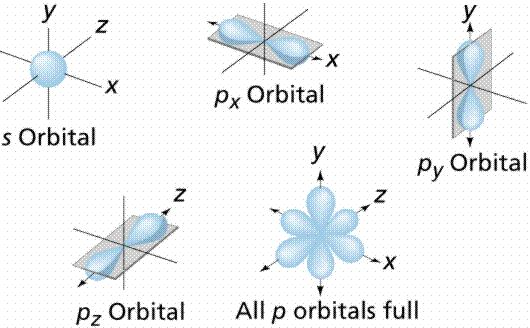Chemistry

# Define Orbital

An orbital is the region of space around the nucleus within which the probability of finding an electron of given energy is maximum. The shape of this region (electron cloud) gives the shape of the orbital. The plot of angular wave functions or square of angular wave functions (probability functions) give us the shapes of orbitals. These two plots differ only slightly. The usually filled orbitals are s, p, d, and f. S orbitals have no pointed nodes and are circular.Example:  The electrons in the 2px orbital of an atom are usually found within a dumbbell-shaped cloud about the x-axis